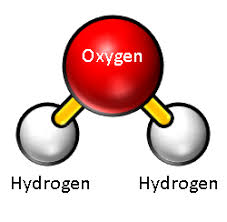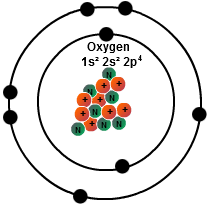# What is Valency of Oxygen & How To Find Valency with Example

Hello friends! We shall discuss what is the valency of oxygenAs you know, valency is the potential of an atom to form a bond with the atom of another element. It is especially the measure of the number of Hydrogen atoms an element can displace or form the bond with.###Oxygen has 8 valence electrons. Hence it belongs to group 6 of the periodic table. This means it has a total of 2 electrons in the first shell (or K shell), and 6 electrons in the first shell (or K shell). Hence it has a valency of 6.

###When oxygen atom forms a covalent bond with two Hydrogen atoms, it forms water (or H2O.)  An oxygen atom contains 8 electrons that revolve around two orbital shells. The first shell, or the inner shell, contains 2 electrons. Whereas, the outer shell contains 6 electrons.

Hydrogen has only one electron which revolves around the proton in a single orbital. Hence both the atoms of the elements are unstable.

To achieve an inert gas electronic configuration or an octet, the oxygen atom must contain 2 more electrons in its outer shell. So to reach this stage, it forms a covalent bond with two Hydrogen atoms to form a covalent bond.Hence the valency of oxygen in water is 2 since it shares two electrons with Hydrogen to reach the inert gas octet electronic configuration.

## One comment

1.Steve Zimmerman says:

There are only 6 valence electrons. Valence electrons are the ones in the outermost shell. The shell has a capacity of 8 which is the maximum for that shell. The fact that an additional 2 electrons could exist in the second shell/ring is how the oxygen atom can bond to other atoms through ionic bonding. The maximum number of electrons which could fill a specific shell is found with the general formula 2(n*n) where n = the shell number. The 3rd shell could have a maximum of 2(3*3) = 2*9 = 18 electrons. The 4th shell …. 2(4*4) = 2*16 = 32. Also the sum of the number of electrons in all of the shells of and atom is equal to the number of protons. This gives the atom a net charge of zero. Gaining or losing an electron(s) results in a positive (+) or negative (-) ion.